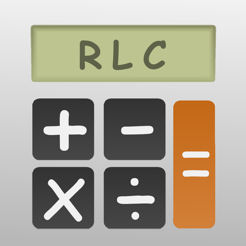## Screenshots

•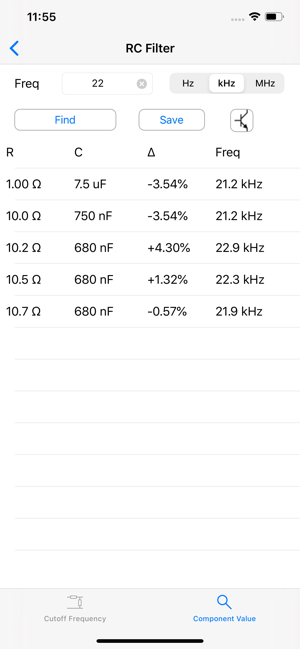•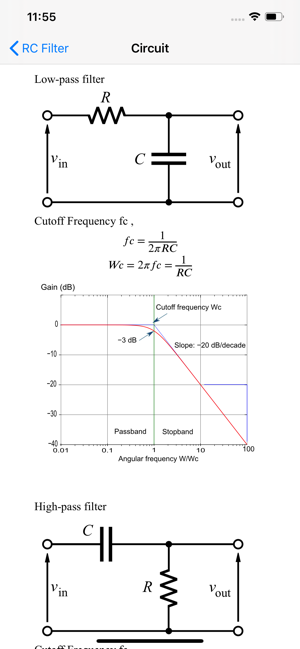•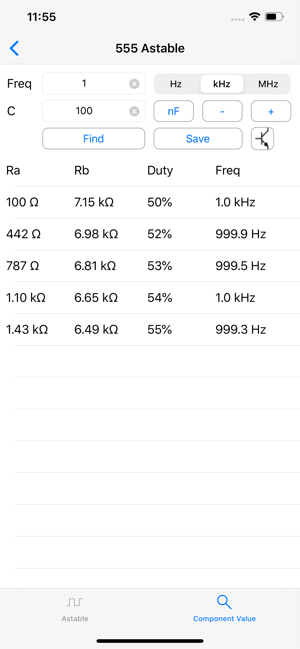•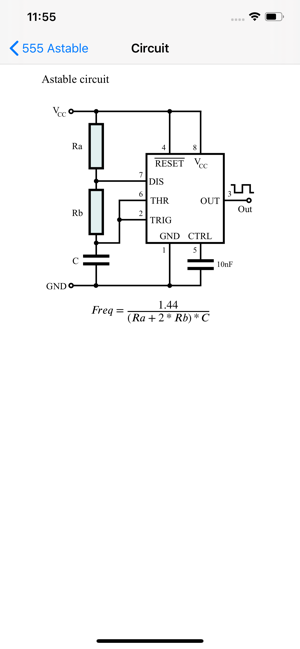•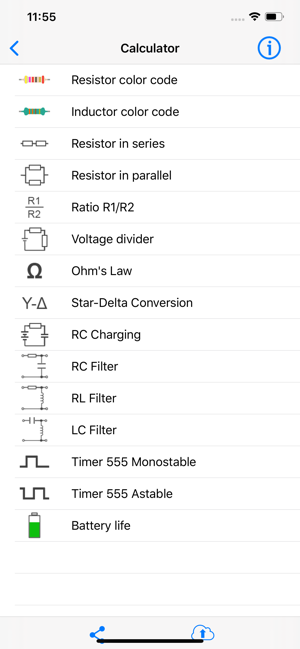## Description

This app is a collection of basic electronic calculators. It is suitable for hobbyist, electronic engineers or professionals.

Features
• Calculate resistance of 2 resistors in series / parallel
• To find out combinations of 2 resistors in series / in parallel making a desired resistance
• Calculate ratio of voltage divider and find out combinations of resistor making a desired ratio
• Calculate inverting amplifier gain and find out combinations of resistor making a desired ratio
• Ohm's Law calculation
• Star-delta conversion
• RC charging circuit : Calculate time constant by R and C
• RC Filter : Calculate cutoff frequency by resistor and capacitor value and find out combinations of resistance and capacitance making a desired cutoff frequency
• RL Filter : Calculate cutoff frequency by resistor and inductor value and find out combinations of resistance and inductance making a desired cutoff frequency
• LC Filter : Calculate resonant frequency by capacitor and inductor values and find out combinations of capacitance and inductance making a desired resonant frequency
• Calculate monostable circuit
• Calculate frequency of astable circuit and find out combinations of capacitor and resistor making a desired astable frequency
• Save combinations in CSV file
* Upload generated CSV files via AirDrop or iCloud Drive

Version 2.9.6

Fix minor bugs

5.0 out of 5
2 Ratings

2 Ratings

## Information

Seller
Siu Yuen Ho
Size
7.5 MB
Category
Utilities
Compatibility

Requires iOS 8.0 or later. Compatible with iPhone, iPad, and iPod touch.

Languages

English

Age Rating
4+
Price
Free
In-App Purchases
1. Unlock Pro features \$1.99

## Supports

•